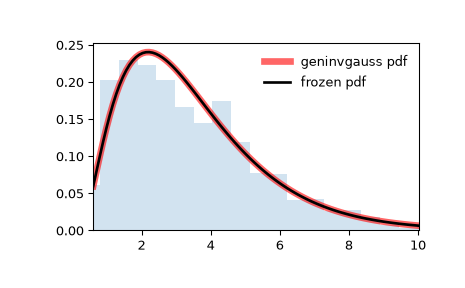# scipy.stats.geninvgauss¶

scipy.stats.geninvgauss(*args, **kwds) = <scipy.stats._continuous_distns.geninvgauss_gen object>[source]

A Generalized Inverse Gaussian continuous random variable.

As an instance of the rv_continuous class, geninvgauss object inherits from it a collection of generic methods (see below for the full list), and completes them with details specific for this particular distribution.

Notes

The probability density function for geninvgauss is:

$f(x, p, b) = x^{p-1} \exp(-b (x + 1/x) / 2) / (2 K_p(b))$

where x > 0, and the parameters p, b satisfy b > 0 (). $$K_p$$ is the modified Bessel function of second kind of order p (scipy.special.kv).

The probability density above is defined in the “standardized” form. To shift and/or scale the distribution use the loc and scale parameters. Specifically, geninvgauss.pdf(x, p, b, loc, scale) is identically equivalent to geninvgauss.pdf(y, p, b) / scale with y = (x - loc) / scale.

The inverse Gaussian distribution stats.invgauss(mu) is a special case of geninvgauss with p = -1/2, b = 1 / mu and scale = mu.

Generating random variates is challenging for this distribution. The implementation is based on .

References

1

O. Barndorff-Nielsen, P. Blaesild, C. Halgreen, “First hitting time models for the generalized inverse gaussian distribution”, Stochastic Processes and their Applications 7, pp. 49–54, 1978.

2

W. Hoermann and J. Leydold, “Generating generalized inverse Gaussian random variates”, Statistics and Computing, 24(4), p. 547–557, 2014.

Examples

>>> from scipy.stats import geninvgauss
>>> import matplotlib.pyplot as plt
>>> fig, ax = plt.subplots(1, 1)


Calculate a few first moments:

>>> p, b = 2.3, 1.5
>>> mean, var, skew, kurt = geninvgauss.stats(p, b, moments='mvsk')


Display the probability density function (pdf):

>>> x = np.linspace(geninvgauss.ppf(0.01, p, b),
...                 geninvgauss.ppf(0.99, p, b), 100)
>>> ax.plot(x, geninvgauss.pdf(x, p, b),
...        'r-', lw=5, alpha=0.6, label='geninvgauss pdf')


Alternatively, the distribution object can be called (as a function) to fix the shape, location and scale parameters. This returns a “frozen” RV object holding the given parameters fixed.

Freeze the distribution and display the frozen pdf:

>>> rv = geninvgauss(p, b)
>>> ax.plot(x, rv.pdf(x), 'k-', lw=2, label='frozen pdf')


Check accuracy of cdf and ppf:

>>> vals = geninvgauss.ppf([0.001, 0.5, 0.999], p, b)
>>> np.allclose([0.001, 0.5, 0.999], geninvgauss.cdf(vals, p, b))
True


Generate random numbers:

>>> r = geninvgauss.rvs(p, b, size=1000)


And compare the histogram:

>>> ax.hist(r, density=True, histtype='stepfilled', alpha=0.2)
>>> ax.legend(loc='best', frameon=False)
>>> plt.show()Methods

 rvs(p, b, loc=0, scale=1, size=1, random_state=None) Random variates. pdf(x, p, b, loc=0, scale=1) Probability density function. logpdf(x, p, b, loc=0, scale=1) Log of the probability density function. cdf(x, p, b, loc=0, scale=1) Cumulative distribution function. logcdf(x, p, b, loc=0, scale=1) Log of the cumulative distribution function. sf(x, p, b, loc=0, scale=1) Survival function (also defined as 1 - cdf, but sf is sometimes more accurate). logsf(x, p, b, loc=0, scale=1) Log of the survival function. ppf(q, p, b, loc=0, scale=1) Percent point function (inverse of cdf — percentiles). isf(q, p, b, loc=0, scale=1) Inverse survival function (inverse of sf). moment(n, p, b, loc=0, scale=1) Non-central moment of order n stats(p, b, loc=0, scale=1, moments=’mv’) Mean(‘m’), variance(‘v’), skew(‘s’), and/or kurtosis(‘k’). entropy(p, b, loc=0, scale=1) (Differential) entropy of the RV. fit(data) Parameter estimates for generic data. See scipy.stats.rv_continuous.fit for detailed documentation of the keyword arguments. expect(func, args=(p, b), loc=0, scale=1, lb=None, ub=None, conditional=False, **kwds) Expected value of a function (of one argument) with respect to the distribution. median(p, b, loc=0, scale=1) Median of the distribution. mean(p, b, loc=0, scale=1) Mean of the distribution. var(p, b, loc=0, scale=1) Variance of the distribution. std(p, b, loc=0, scale=1) Standard deviation of the distribution. interval(alpha, p, b, loc=0, scale=1) Endpoints of the range that contains alpha percent of the distribution

#### Previous topic

scipy.stats.genhalflogistic

#### Next topic

scipy.stats.gilbrat